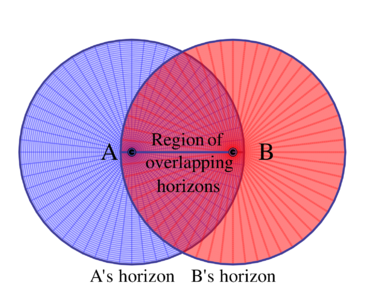# Simple English

(diff) ← Older revision | Latest revision (diff) | Newer revision → (diff)

A vague definition of a horizon can be accepted as the following: it is a frontier between things observable and things unobservable.

Particle horizon.. If the Universe has a finite age, then light travels only a finite distance in that time and the volume of space from which we can receive information at a given moment of time is limited. The boundary of this volume is called the particle horizon.

Event horizon. The event horizon is the complement of the particle horizon. The event horizon encloses the set of points from which signals sent at a given moment of time will never be received by an observer in the future.

Space-time diagram is a representation of space-time on a two-dimensional plane, with one timelike and one spacelike coordinate. It is typically used for spherically symmetric spacetimes (such as all homogeneous cosmological models), in which angular coordinates are suppressed.

References:

## Contents

### Problem 1

Draw a space-time diagram that shows behaviour of worldlines of comoving observers in a

1. stationary universe with beginning
2. expanding universe in comoving coordinates
3. expanding universe in proper coordinates

### Problem 2

Suppose there is a static universe with homogeneously distributed galaxies, which came into being at some finite moment of time. Draw graphically the particle horizon for some static observer.

### Problem 3

How does the horizon for the given observer change with time?

### Problem 4

Is there an event horizon in the static Universe? What if the Universe ends at some finite time?

### Problem 5

The horizon riddle. Consider two widely separated observers, A and B (see Figure). Suppose they have overlapping horizons, but each can apparently see things that the other cannot. We ask: Can B communicate to A information that extends A's knowledge of things beyond his horizon? If so, then a third observer C may communicate to B information that extends her horizon, which can then be communicated to A. Hence, an unlimited sequence of observers B, C, D, E,... may extend A's knowledge of the Universe to indefinite limits. According to this argument A has no true horizon. This is the horizon riddle. Try to resolve it for the static Universe.The horizon riddle: can two observers with overlapping horizons pass information to each other regarding things outside of the other's horizon?

### Problem 6

Suppose observer O in a stationary universe with beginning sees A in some direction at distance $L$ and B in the opposite direction, also at distance $L$. How large must $L$ be in order for A and B to be unaware of each other's existence at the time when they are seen by O?

### Problem 7

Draw spacetime diagrams in terms of comoving coordinate and conformal time and determine whether event or particle horizons exist for:

• the universe which has a beginning and an end in conformal time. The closed Friedman universe that begins with Big Bang and ends with Big Crunch belongs to this class.
• the universe which has a beginning but no end in conformal time. The Einstein--de Sitter universe and the Friedman universe of negative curvature, which begin with a Big Bang and expand forever, belong to this class.
• the universe which has an end but no beginning in conformal time. The de Sitter and steady-state universes belong to this class.
• the universe which has no beginning and no ending in conformal time. The Einstein static and the Milne universes are members of this class.

Conformal time is the altered time coordinate $\eta=\eta (t)$, defined in such a way that lightcones on the spacetime diagram in terms of $\eta$ and comoving spatial coordinate are always straight diagonal lines, even when the universe is not stationary.

### Problem 8

Draw the spacetime diagram in terms of comoving space and ordinary time or the universe with an end but no beginning in conformal time.

### Problem 9

Formulate the necessary conditions in terms of conformal time for a universe to provide a comoving observer with

• a particle horizon
• an event horizon

### Problem 10

Consider two galaxies, observable at present time, $A$ and $B$. Suppose at the moment of detection of light signals from them (now) the distances to them are such that $L_{det}^{A}<L^{B}_{det}$. In other words, if those galaxies had equal absolute luminosities, the galaxy $B$ would seem to be dimmer. Is it possible for galaxy $B$ (the dimmer one) to be closer to us at the moment of its signal's emission than galaxy $A$ (the brighter one) at the moment of $A$'s signal's emission?

### Problem 11

Show on a spacetime diagram the difference in geometry of light cones in universes with and without particle horizons.